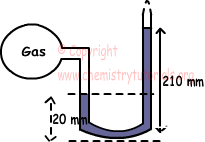EN | ES | DE | NL | RU

Gases Exam2 and Problem Solutions

Gases Exam2 and  Problem Solutions

1. Find value of 570 mm Hg in terms of atm.

Solution:

We know that there is a relation between atm and cm Hg;

1 atm = 76 cm Hg =760 mm Hg

760 mm Hg is 1 atm

570 mm Hg is ? atm

¯¯¯¯¯¯¯¯¯¯¯¯¯¯¯¯¯

?=0,75 atm

2. Atmospheric pressure is 1 atm. Find pressure of gas in terms of atm;

I. If system is closed manometer

II. If system is open manometer.Solution:

I. If system is closed;

Pgas=210-20=190 mm Hg

760 mm Hg is 1 atm

190 mm Hg is ? atm

¯¯¯¯¯¯¯¯¯¯¯¯¯¯¯¯¯

?=0,25 atm

II. If system is opened;

Pgas=Pair + (210-20)mm Hg

Pair=1 atm=760 mm Hg

Pgas=760 +190 = 950 mm Hg =95 cm Hg

3. Pressure of gas having 1 liter volume is  380 mm Hg. If volume is decreased to 200 cm3, find change in the pressure under constant temperature.

Solution:

Pi=380 mm Hg

Vi=1lt=1000 cm3

Vf=200 cm3

Pf=?

We use boyle's law;

Pi.Vi=Pf.Vf

380.1000=Pf.200

Pf=1900 mm Hg=2,5 atm

4. Find pressure of gas under 546 0C, that has pressure 200 mm Hg under 273 0C.

Solution:

Pi=200 mmHg, Pf=?, Ti=273 0C, Tf=546 0C

We use Guy Lussac Law;

Pi/Ti=Pf/Tf

But, we should first convert temperatures from 0C to 0K.

Ti=273 + 273 = 546 0K

Tf= 546 + 273 = 819 0K

200/546=Pf/819

Pf=300 mmHg

5. Find pressure of CO2 having 8,8 g mass and 1230 cm3 volume under 27 0C temperature. (CO2=44)

Solution:

mole of CO2 =mass/molar mass=8,8/44=0,2mol

T=27 + 273=300 0K

V=1230 cm3 =1,23 liter

Ideal gas law is used;

P.V=n.R.T

P.1,23=0,2.0,082.300

P=4 atm

The Original Author: